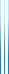# PublicationsManuscripts
## On the sequence $n$ mod $x$
Avinoam Braverman considered the sequence $n \bmod x$, where $1 \leq x \leq \sqrt{n}$, took its local minima, and plotted the results. When $n$ is large, a curious pattern composed out of what seem to be triangles appears. We explain this phenomenon heuristically, given formulas for the envelope of the “triangles” (which turn out to be quadratic functions). We go on to describe the envelope of the plot when an arbitrary number of the local minima and local maxima operations are composed.